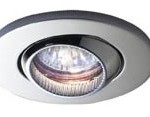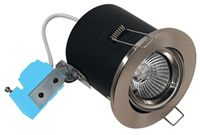# Low voltage Lighting

See what our customers had to say about our work with Low Voltage LightingÂ here,Â here,Â here,Â andÂ here.

Is low voltage the same as low energy?

No! It’s the watts that count, not the volts.There is a common misconception that low voltage lighting systems are the same thing in terms of energy efficiency as low energy lighting systems. On this page we will try to explain why this is.

Measuring energy
Energy is measured in watts – your electricity bill probably shows how many kilowatts you have used. A kilowatt is 1000 watts.

Therefore, if you can produce a lot of light while using a small amount of watts you have a low energy light, and a cheaper electricity bill.

You probably know that low energy light bulbs have a small wattage rating and are often compared to an equivalent wattage. You might see that an 11w low energy bulb is the equivalent of a 60w normal bulb. This is only comparing the amount of light that is produced, it has nothing to do with the amount of energy consumed.

Volts, amps and watts
To show that a low voltage light is not a low energy light, we will compare these three lights:• 50w low voltage spot light
• 50w mains voltage spot light
• 9w low energy spot light.

All three examples will produce about the same amount of light, but only one will cost less to run.

You might remember from your school days that watts = volts x amps. Once we know this we can easily show that the maths confirms the number of watts used by each of the three example light:

 Volts(The electric supplyconnected to the light) Amps(watts dividedby volts) Watts(as described bythe product) 50w low voltage spot light 12 4.17 50 50w mains voltage spot light 240 0.21 50 9w low energy spot light 240 0.03 9

But doesn’t it use less power because it’s running at 12 volts?
No – watts are watts. It doesn’t matter what the voltage is. We can show this more clearly by explaining about transformers: